## Cognitive Study (First Update)¶

### Elan Ding¶

Modified: Aug 13, 2018

I have combined all data into a single csv file called cognition.csv.

In :
import pandas as pd
import numpy as np
import matplotlib.pyplot as plt
import seaborn as sns

In :
df = pd.read_csv('cognition2.csv')


The dataset cognition2.csv is an udpated version of the previous cognition.csv. Mostly we included more data for the second visit. To get an idea, let's see a countplot.

In :
plt.rcParams['figure.figsize'] = (10, 8)
sns.countplot(df['Visit'].astype(str), data=df)

Out:
<matplotlib.axes._subplots.AxesSubplot at 0x10efe0b70>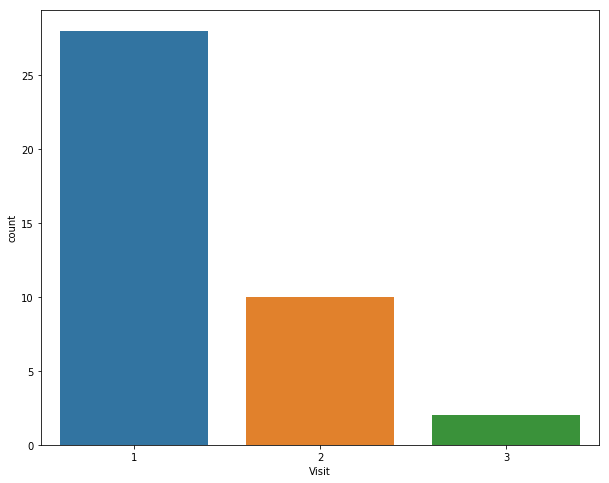## Exploratory Data Analysis¶

### Objective 1: Plotting cognitive scores and total QOL scores stratified by disease (MM vs Control).¶

In :
sns.lmplot(x='Cog Score', y='Total Score', hue='Disease', data=df, size=8)

Out:
<seaborn.axisgrid.FacetGrid at 0x10ef86e48>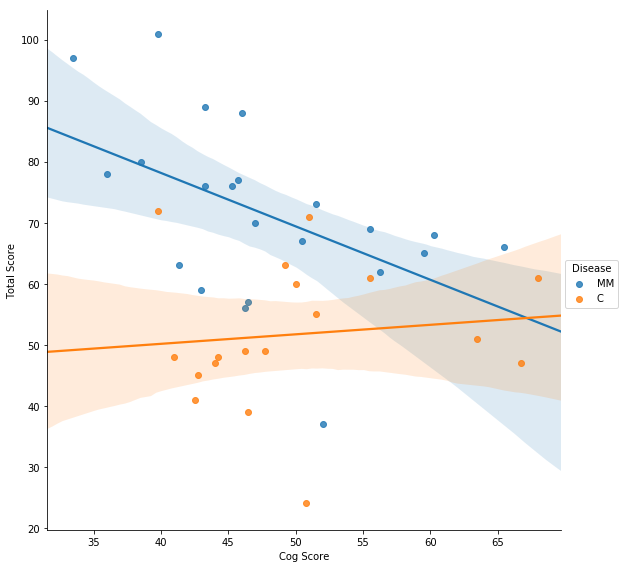It looks like that among MM patients, the cog score is inversely related to the QOL scores. For the control the relationship is opposite. This makes sense.

### Objective 2: Does sequence affect cognitive scores?¶

In :
sns.boxplot(x = 'Sequence', y='Cog Score', data=df)

Out:
<matplotlib.axes._subplots.AxesSubplot at 0x114372c88>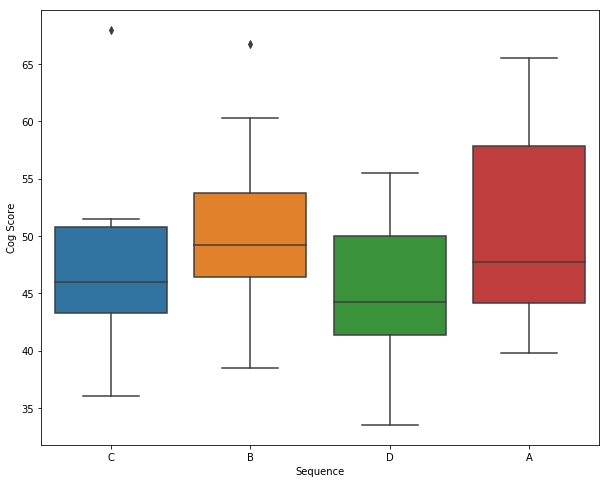Looks like that sequence A gives higher score. This is just a minor observation.

### Objective 3: Is gender a significant factor?¶

In :
sns.countplot(df['Gender'].astype(str), data=df)

Out:
<matplotlib.axes._subplots.AxesSubplot at 0x114bb2898>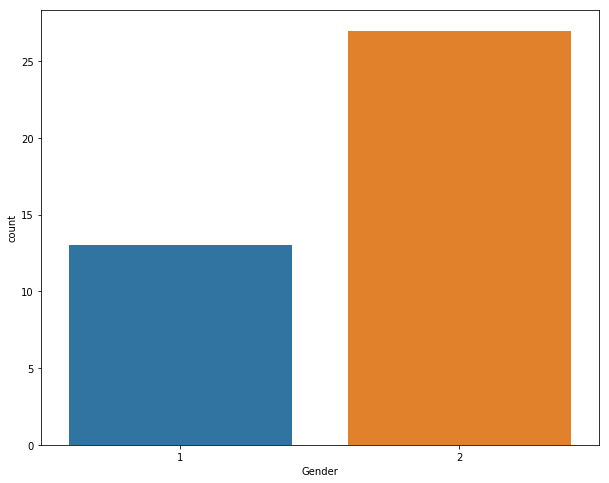In :
sns.boxplot(x = 'Gender', y='Cog Score', data=df)

Out:
<matplotlib.axes._subplots.AxesSubplot at 0x114be9320>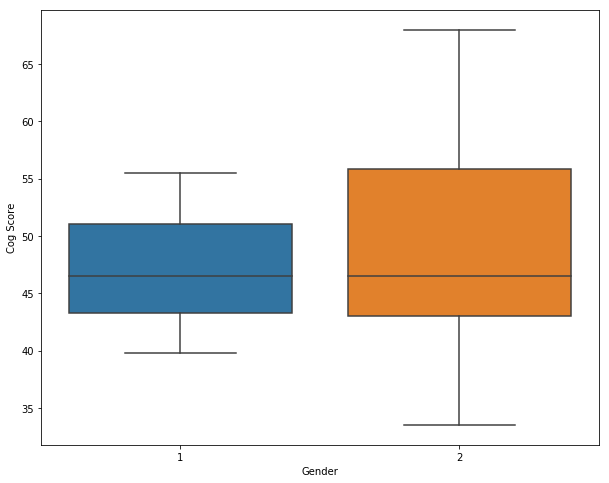In :
sns.boxplot(x = 'Gender', y='Cog Score', hue='Disease', data=df)

Out:
<matplotlib.axes._subplots.AxesSubplot at 0x114d78748>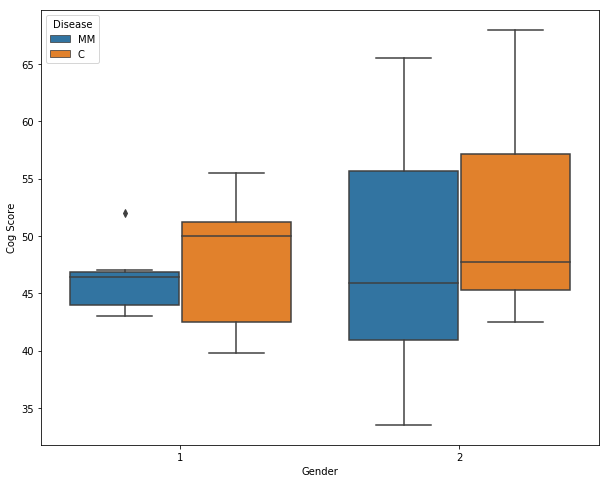In :
sns.boxplot(x = 'Gender', y='Total Score', data=df)

Out:
<matplotlib.axes._subplots.AxesSubplot at 0x114f39668>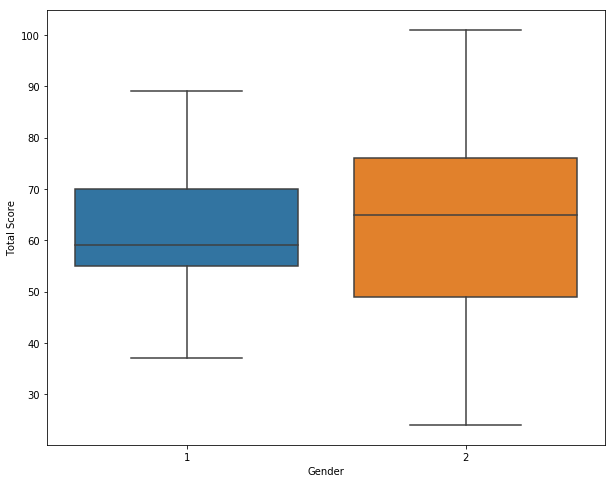It appears that gender is not a very significant factor.

### Objective 4: Is there a difference between MM patients and control?¶

In :
sns.countplot(df['Disease'].astype(str), data=df)

Out:
<matplotlib.axes._subplots.AxesSubplot at 0x1150ca198>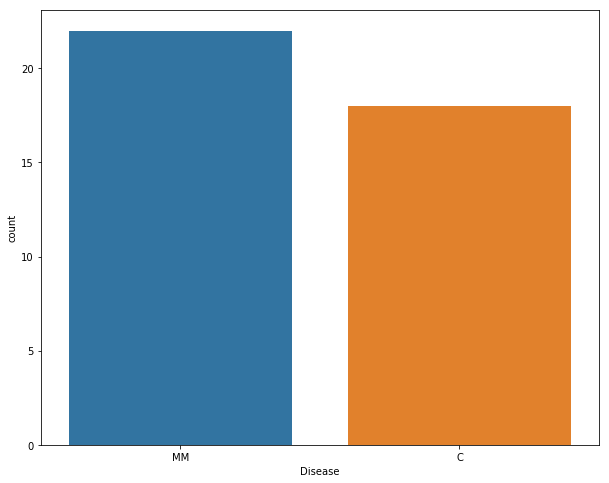In :
sns.boxplot(x = 'Disease', y='Cog Score', data=df)

Out:
<matplotlib.axes._subplots.AxesSubplot at 0x11510e0b8>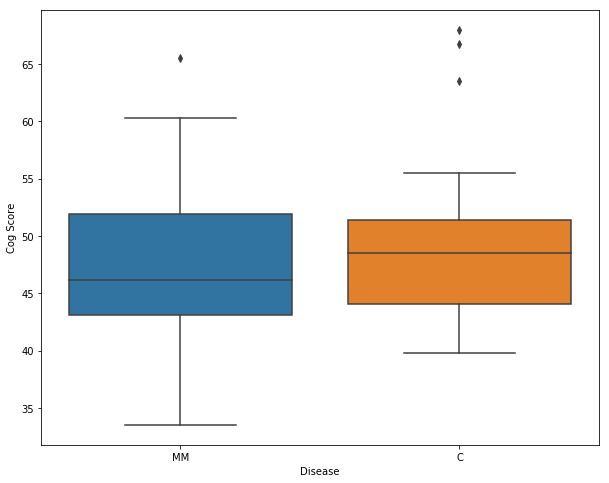In :
sns.boxplot(x = 'Disease', y='Cog Score', hue='Visit', data=df)

Out:
<matplotlib.axes._subplots.AxesSubplot at 0x1a1bf5fb70>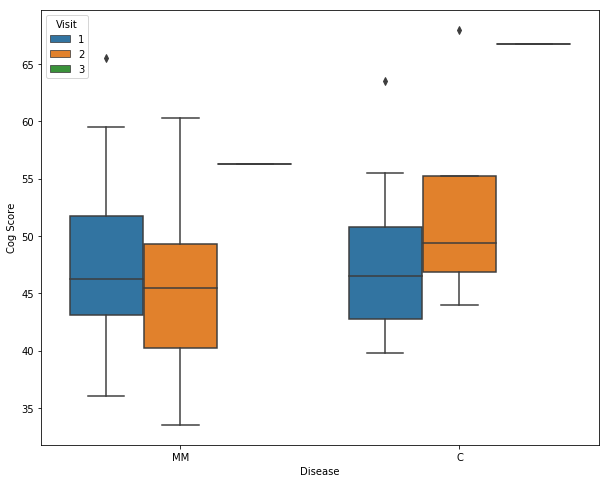Here we observe that among MM patients, the second visit gives a lower cognitive score, while among the controls, the second visit produced a higher score. Update: The trend remains; however, as we have more data for the second visit, the decrease in cog score among MM patients appears to be weaker than before.

### Statistical Analysis for Objective 4¶

Although we see a slight decrease in cog scores among the MM patients and slight increase in cog scores among the control, they are not statistically significant at this point.

In :
visit1_MM = df[(df['Disease']=='MM') & (df["Visit"]==1)]["Cog Score"]
visit2_MM = df[(df['Disease']=='MM') & (df["Visit"]==2)]["Cog Score"]

visit1_C = df[(df['Disease']=='C') & (df["Visit"]==1)]["Cog Score"]
visit2_C = df[(df['Disease']=='C') & (df["Visit"]==2)]["Cog Score"]

print("Among the MM group, there are {} first visits, with mean equal to {}.".format(len(visit1_MM),np.mean(visit1_MM)))
print("Among the MM group, there are {} second visits, with mean equal to {}.".format(len(visit2_MM), np.mean(visit2_MM)))
print("Among the C group, there are {} first visits, with mean equal to {}.".format(len(visit1_C), np.mean(visit1_C)))
print("Among the C group, there are {} second visits, with mean equal to {}.".format(len(visit2_C), np.mean(visit2_C)))

Among the MM group, there are 15 first visits, with mean equal to 47.75555555533333.
Among the MM group, there are 6 second visits, with mean equal to 45.625.
Among the C group, there are 13 first visits, with mean equal to 47.96153846153846.
Among the C group, there are 4 second visits, with mean equal to 52.6875.

In :
from scipy.stats import ttest_ind as ttest

t_MM, p_MM = ttest(visit1_MM, visit2_MM)
t_C, p_C = ttest(visit1_C, visit2_C)

print("Comparing cog scores between two visits among the MM group, the p-value is {}.".format(p_MM))
print("Comparing cog scores between two visits among the MM group, the p-value is {}.".format(p_C))

Comparing cog scores between two visits among the MM group, the p-value is 0.5993131238028242.
Comparing cog scores between two visits among the MM group, the p-value is 0.2904097549666309.

In :
sns.boxplot(x = 'Disease', y='Physical Score', hue='Visit', data=df)

Out:
<matplotlib.axes._subplots.AxesSubplot at 0x1a1c149ba8>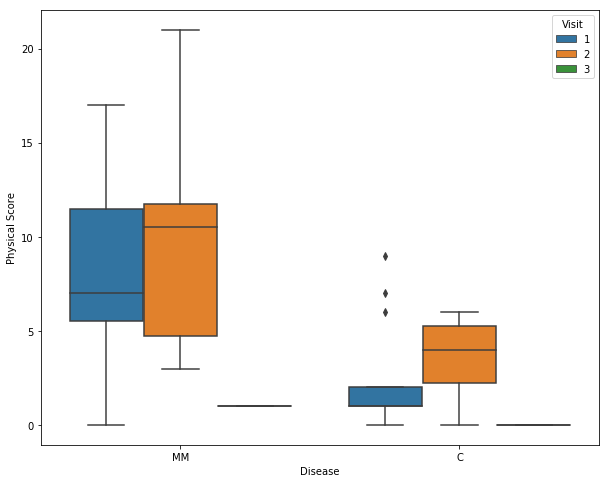In :
sns.boxplot(x = 'Disease', y='Social Score', data=df)

Out:
<matplotlib.axes._subplots.AxesSubplot at 0x1a1c321e10>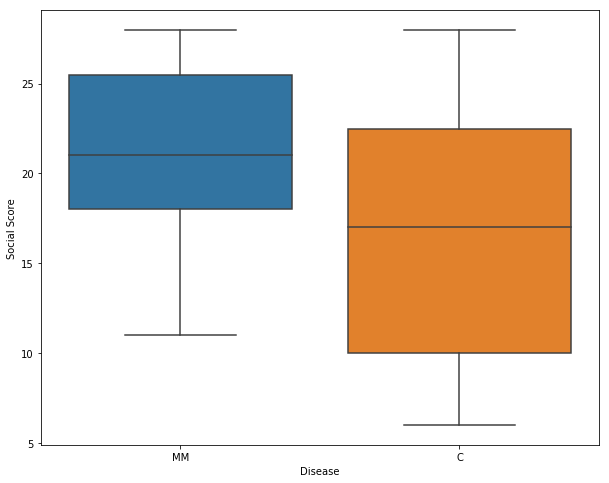In :
sns.boxplot(x = 'Disease', y='Social Score', hue='Visit', data=df)

Out:
<matplotlib.axes._subplots.AxesSubplot at 0x1a1c4a9940>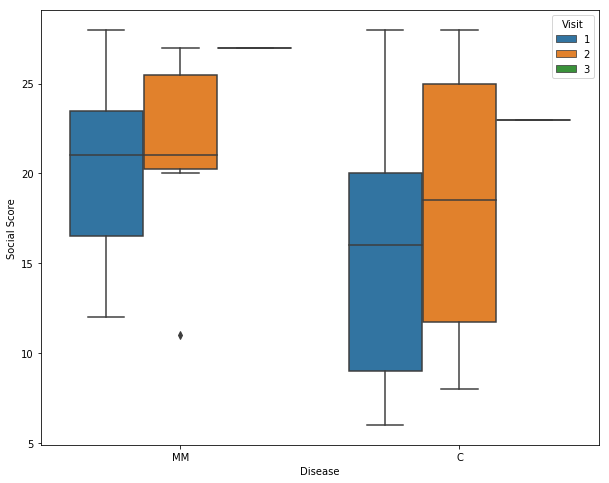In :
sns.boxplot(x = 'Disease', y='Economic Score', data=df)

Out:
<matplotlib.axes._subplots.AxesSubplot at 0x1a1c688ba8>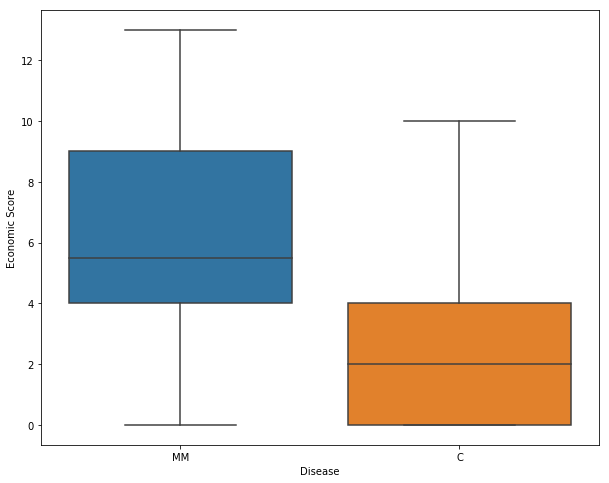In :
sns.boxplot(x = 'Disease', y='Economic Score', hue='Visit', data=df)

Out:
<matplotlib.axes._subplots.AxesSubplot at 0x1a1c8147f0>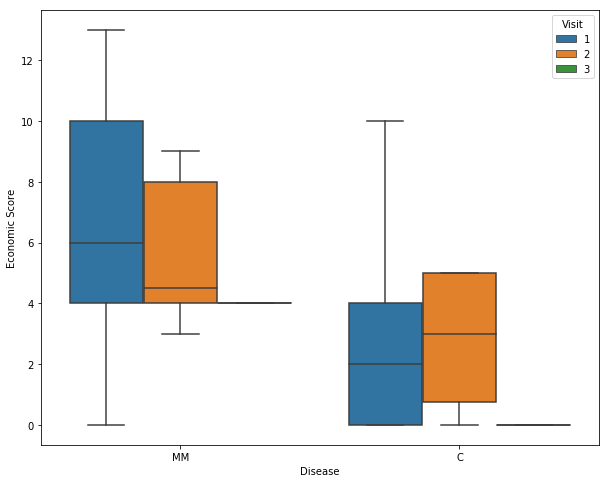In :
sns.boxplot(x = 'Disease', y='Functional Score', data=df)

Out:
<matplotlib.axes._subplots.AxesSubplot at 0x1a1c9facc0>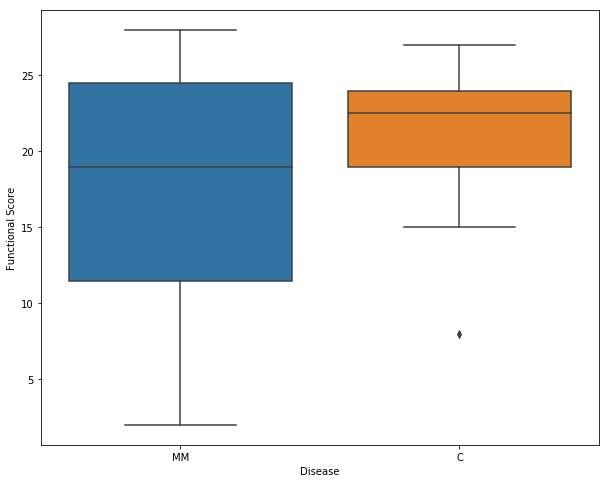In :
sns.boxplot(x = 'Disease', y='Functional Score', hue='Visit', data=df)

Out:
<matplotlib.axes._subplots.AxesSubplot at 0x1a1cb86940>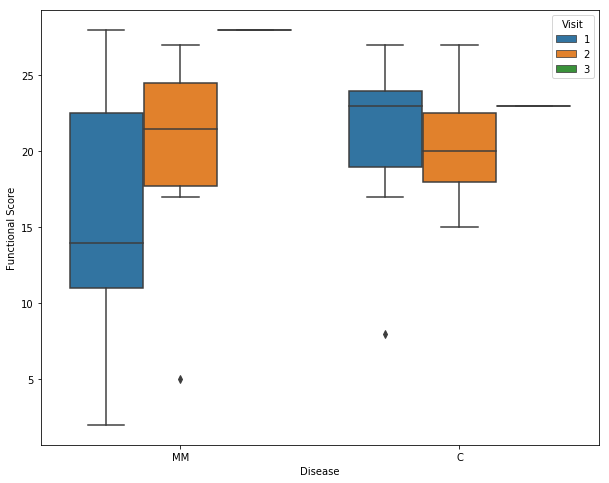In :
sns.boxplot(x = 'Disease', y='Additional Score', data=df)

Out:
<matplotlib.axes._subplots.AxesSubplot at 0x1a1cd5a7b8>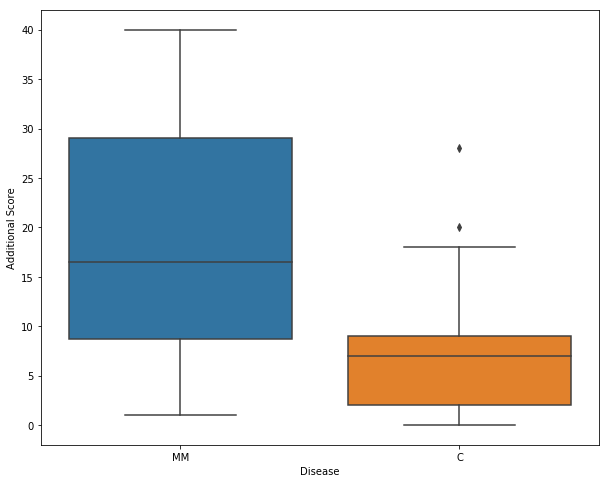In :
sns.boxplot(x = 'Disease', y='Additional Score', hue='Visit', data=df)

Out:
<matplotlib.axes._subplots.AxesSubplot at 0x1a1cef4e10>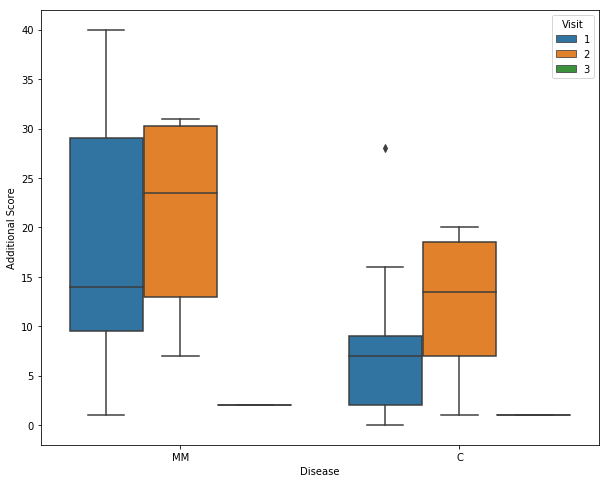In :
sns.boxplot(x = 'Disease', y='Total Score', data=df)

Out:
<matplotlib.axes._subplots.AxesSubplot at 0x1a1d0d0080>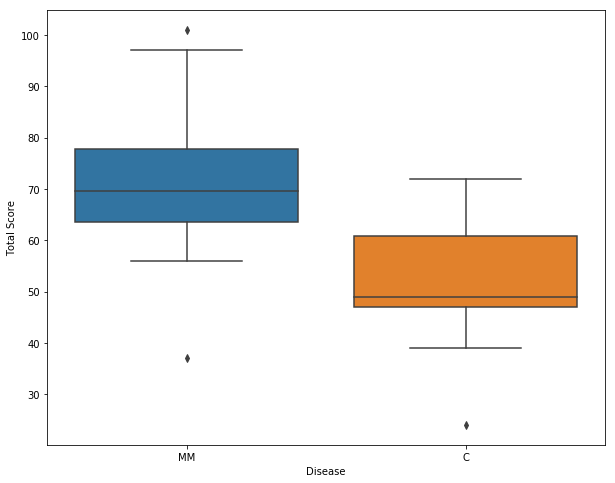In :
sns.boxplot(x = 'Disease', y='Total Score', hue='Visit', data=df)

Out:
<matplotlib.axes._subplots.AxesSubplot at 0x1a1d262588>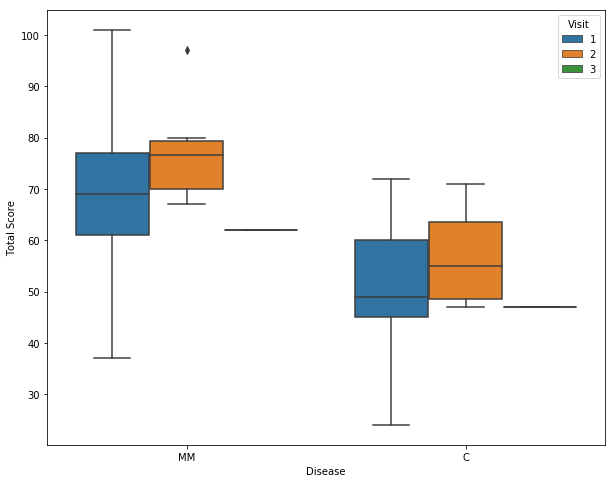Interestingly, we see an improvement between first and second visits in both MM and control groups.